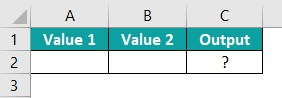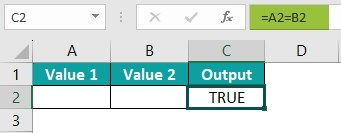# Compare Two Columns in Excel for MatchesArticle byExcelMojo Team## What Is Compare Two Columns In Excel For The Matches?

The Compare Two Columns in Excel function is a powerful tool that allows users to quickly and easily identify matches and differences between two data sets. This feature finds items that appear in both columns, enabling comparisons and data analysis. Matched values are highlighted in the output, making it simple to see which entries from each set of data correspond with one another.

In the following example, we will compare two Excel columns using an Excel formula. This process will allow us to efficiently analyze and identify discrepancies or similarities between the two columns. By implementing this technique, we can streamline our data analysis and make informed decisions based on accurate information.

The outcome obtained by utilizing the formula =A2=B2 is displayed in the image below.

###### Key Takeaways
• The methods efficiently identify potential data issues and discrepancies between two data sets within Excel spreadsheets.
• The function can save significant time and resources when performing valuable data analysis tasks within an Excel spreadsheet.
• Comparing two columns can also be useful when consolidating data from multiple sources, ensuring accuracy while minimizing errors and reducing manual efforts required to maintain data quality standards.
• Various factors make it a valuable tool for professionals looking to analyze large datasets quickly and accurately while gaining meaningful insights into their business operations.

### How To Compare Two Columns In Excel For The Matches?

When needing to compare two columns for matches and differences in Excel, there are several pathways one can take.

One method is to use the conditional formatting feature in Excel. Here, we can select both columns, head to the ‘’Home’’ tab and click on Conditional Formatting > Highlight Cells Rules > Duplicate Values. This will highlight any matching cell values in both columns.

Another option is using formulas such as VLOOKUP or COUNTIF excel function to automate the comparison process. For instance, a formula can be created in a third column displaying “Yes” if a match is found or “No” where no match exists.

Finally, adding filters to each column and sorting them alphabetically may assist with comparing each column side by side, as matches will be easier to spot when visually aligned.

#### Example #1 – Simple Method

In the following example, numerical values are displayed. We will compare two Excel columns by utilizing an Excel formula. This process will enable us to analyze and identify discrepancies or similarities between the two columns. By implementing this technique, we can streamline our data analysis and make informed decisions based on accurate information.

In the table,

• Columns A, B, and C shows value 1, value 2 and the output

To calculate the value using the Excel Formula, follow these steps:

Step 1: Choose the cell where the formula will be entered, and the result will be calculated. For this example, we will select cell C2.

Step 2: Enter the complete formula =A2=B2 in cell C2.

Step 3: Press the Enter key. The resulting value in cell C2 should be TRUE, as depicted in the image below. To proceed, press Enter and drag the cursor to cell C6, as illustrated in the following image.

#### Example #2 – Match Case Sensitive Values

In the following example, we will showcase using string values to compare two Excel columns using the EXACT excel function for matching Case Sensitive Values. We can analyze and identify discrepancies or similarities between the two columns by implementing an Excel formula. This process is crucial in streamlining our data analysis and making informed decisions based on accurate information.

In the table

• Columns A, B, and C shows value 1, value 2, and output

To calculate the value using the Excel Formula, follow these simple steps:

Step 1: Select the cell where the formula will be entered, and the result will be calculated. For this example, we will be selecting cell C2.

Step 2: Input the entire formula =EXACT (A2, B2) into cell C2.

Step 3: Press the Enter key. The resulting value in cell C2 should be FALSE, as shown in the image below. To continue, press Enter and drag the cursor to cell C5, as demonstrated in the subsequent image.

It is imperative to follow these steps to ensure the desired outcome accurately. The program will calculate the values and display the result in cell C2 by inputting the formula and pressing Enter. This will confirm that the formula has been entered correctly. Once verified, proceed to the next step by pressing Enter and dragging the cursor to cell C5. This will allow the formula to be applied to the desired range of cells.

### Important Things To Note

• Excel’s Compare Two Columns lets users modify the settings for more customizable results.
• Case sensitivity can be toggled on or off, allowing text-based matches regardless of capitalization.
• Comparison operators such as “equal to,” “greater than,” and “less than” enable more stringent criteria for value matching beyond textual similarities.
• Comparing two columns for matches is a useful tool that allows us to logically compare the values of two different cells to determine if they are similar or different. The output of this comparison is always a Boolean value, either TRUE or FALSE.

1. What happens if there are no matches between the two columns in Excel?

If there are no matches between the two Excel columns, the values on one column do not correspond to any values on the other. This can cause issues when performing calculations or data analysis, as the missing data may affect the accuracy and completeness of the results.
To fix this, we can manually review both columns and identify potential errors or discrepancies that could be causing the mismatch. Another option is to use Excel’s built-in functions, such as VLOOKUP or INDEX/MATCH, to help locate and retrieve missing data from other sources.

2. Why Is It Useful to Compare Two Columns in Excel?

Comparing two columns in Excel is useful to identify and detect discrepancies or duplications in data. It determines trends and patterns by highlighting similarities and differences between the data sets.

For example, we will compare two columns in Excel to analyze and identify discrepancies or similarities between the two columns.The result achieved by utilizing the formula =A2=B2 is exhibited in the image below.3. What are the other methods to compare two columns in Excel using the IF condition?

One uses VLOOKUP and returns a match if one column exists in the other.
We can also use COUNTIF to tally how many times a value appears in one column and see if it does or does not exist in another column.
Lastly, we can use the MATCH function alongside an IF condition within an array formula to retrieve either “Match” or “No Match” results based on conditions specified for both columns.